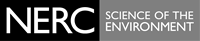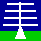THE NERC MST RADAR FACILITY AT ABERYSTWYTH

# File format for MST Radar spectral data

spectra, for a single dwell, with Power Spectral Densities (PSDs) given in dB units. The latter are equal to 10.0 * log10(PSD_linear_units). Click here to find out more about the spectral level processing. Each dwell may contain data for ST-mode observations (which cover the approximate altitude range 2 - 20 km) or for M-mode observations (which cover the approximate altitude range 58 - 94 km) or for both together. The spectra require dc correction. For spectra derived using a Rectangular data weighting window, this requires the PSD of the zero-Doppler-velocity velocity bin to be replaced with a value linearly interpolated between the PSDs at adjacent velocity bins. This correction must be extended to cover the central three velocity bins in the case of spectra derived using a Hanning window. An overview of the radar measurement technique and of the meanings of the data products is given here.

Availability
MST radar spectral data are currently only available in this format starting on6th February 2007. Data from earlier dates are available in a legacy format.

File naming convention:
Files containing spectra for a single dwell are named with the format:

where YYYYMMDDhhmmss is a string of 14 (numerical) characters representing a start date-time (i.e. year-month-day-hour-minute-second). The first of these strings represents the start date-time of the relevant cycle of observation and the second represents the start date-time of the dwell.

All spectral files with cycle start date-times within a 30 minute block (starting at 00 and 30 minutes past each hour) are combined into a single tar file with the naming convention:

where YYYYMMDD_hhmm represents the start date-time of the block. These are the files available through the BADC. The individual spectral files can be extracted on a Linux/Unix platform using the command:

A tool such as WinZip must be used to extract the files on a MS Windows platform.

Archiving convention: YYYY/MM/DD

netCDF File Structure using the file for 2007/02/06 14:05:10 as an example- click here for an explanation
List of Global attributes - Click on the name to view the value
char dwell_id
char cycle_id
char dwell_format_file_path
char history
char version
char institution
char source
char references
List of Dimensions - Click on the name to view the corresponding coordinate variable
range
doppler_velocity
List of Variables - Click on a name to view the corresponding attributes
float range (range)
float doppler_velocity (doppler_velocity)
float power_spectral_density (range, doppler_velocity)
byte length_of_transmitter_pulse ()
byte sub_length_of_transmitter_pulse ()
short bottom_range_gate_number_for_ST_mode ()
short top_range_gate_number_for_ST_mode ()
short bottom_range_gate_number_for_M_mode ()
short top_range_gate_number_for_M_mode ()
short number_of_coherent_integrations ()
short number_of_incoherent_integrations ()
short inter_pulse_period ()
short number_of_complex_samples_in_discrete_fourier_transform ()
byte data_weighting_window_index ()
short year ()
short month ()
short day ()
float time ()
byte beam_pointing_direction_number ()
float beam_pointing_azimuth_angle ()
float beam_pointing_zenith_angle ()
integer range_resolution ()
short range_resolution_number ()
List of Global Attribute values
char dwell_id = "20070206140510"

char cycle_id = "20070206140510"

char dwell_format_file_path = "/home/mst/acquire_mst_dwell_formats/dwell-format_st_standard.txt"

char history = "
Tue Feb 6 14:05:10 2007 - /usr/local/bin/acquire_mst_dwell -cycle_id 20070206140510 -dwell_format_filename dwell-format_st_standard.txt -beam_pointing_direction_number 11
"

char version = "0.1"

char institution = "
Data recorded by the Natural Environment Research Council (NERC)
Mesosphere-Stratosphere-Troposphere (MST) Radar Facility at Aberystwyth
- http://mst.nerc.ac.uk
Data processed by the Rutherford Appleton Laboratory, Space Science and
Technology Department - http://www.sstd.rl.ac.uk
Data held at the British Atmospheric Data Centre
"

char source = "
The Natural Environment Research Council (NERC) Mesosphere-Stratosphere-
"

char references = "
Basic information about the data is available at
More detailed information about the data is available at
http://mst.nerc.ac.uk
"

The Natural Environment Research Council (NERC) Mesosphere-Stratosphere-
Troposphere (MST) Radar at Aberystwyth (UK) is a 46.5 MHz
wind-profiling instrument. It transmits short pulses of radio waves which are scattered back to it from atmospheric targets. The distance
of a target from the instrument is determined by the time delay
between the transmission and reception of a pulse. The main targets
are metre-scale refractive index irregularities, which are referred to
as clear-air targets (the term does not necessarily imply clear-sky
conditions as the radar is able to see through clouds). Hydrometeors,
aircraft, and ground-based objects can also give rise to radar
returns. The receiver signal is occasionally contaminated by
interference. The refractive index irregularities are caused by
variations in atmospheric humidity (within the lowest 10 km of the
atmosphere), in atmospheric density (within the lowest few 10s of km)
and in free electron density (above 50 km). The radar return signal
power is typically proportional to the square of the mean vertical
gradient of the (potential) refractive index and inversely
proportional to the square of the range of the clear-air targets from
the radar. The refractive index irregularities are assumed to be
component of the wind vector along the radar beam pointing direction,
can be inferred from the Doppler shift of the radar return
signal. Atmospheric turbulence gives rise to variability in the radial
velocity when observed over a time scale of a few tens of
seconds. However, as a result of the radar\'s finite beam width, the
which is proportional to the horizontal wind speed. Consequently it
becomes increasingly difficult to infer turbulence intensities as the
wind speed increases.
the transmission of a pulse. This corresponds to sampling at 150.0 m
intervals in range from the radar. It is necessary to sample both the
(i.e. complex values) in order to allow both the magnitude and the
sign of the Doppler shift to be inferred. In the m-mode samples are
recorded between ranges of approximately 60 and 90 km. In the st-mode
samples are recorded between ranges of approximately 2and 20 km. It
is possible to operate the radar in mst-mode so that both altitude
ranges are sampled simultaneously. The range resolution of the radar
returns is determined by the length of the transmitter pulse (not by
the sampling interval), to which the receiver bandwidth must be
matched. The range resolution can be increased by using complementary
coding. This requires the phase of sub-lengths of the transmitter
pulse to be offset by either 0 or 180 degrees according to a set
coding pattern. The range resolution is then determined by the
sub-length of the transmitter pulse (to which the receiver bandwidth
is matched).
samples have been acquired for a large, pre-determined number of
pulses - typically covering a few tens of seconds. The term dwell is
used to refer to this collection interval or to range profiles of any
of the data products associated with it. A dwell initially consists of
a complex time series, for each range gate, of I and Q samples which
are separated in time by the inter pulse period (of the order of a
millisecond). The nature of the samples changes only slowly from pulse
to pulse and so coherent integration is applied - for each range gate,
groups of consecutive samples are summed together. The number of
complex samples in the resulting time series is thus reduced, and the
time interval between them is increased, by a factor equal to the
number of coherent integrations (typically of the order of a few
hundred). The time interval between the new samples (typically of the
order of 0.1 s) determines the Nyquist velocity (typically of the
order of 10 m/s), the maximum radial velocity that can be
unambiguously determined. Decoding must be applied to the coherently
integrated samples if a complementary transmitter code has been
used. For each range gate, a complex Doppler frequency spectrum is
derived by applying a weighting window to the complex time series data
followed by a discrete Fourier transform. This spectrum is multiplied
by its complex conjugate to give a power spectrum. Doppler frequencies
are converted into Doppler velocities by multiplying by half the radar
wavelength. The sign must be changed so that movement away from the
radar (which gives rise to a negative frequency shift) is represented
by a positive velocity. If desired, consecutively-observed Doppler
velocity power spectra may be incoherently integrated by adding them
together. This increases the detectability of signals. In general a
Doppler velocity power spectrum contains one or more signal components
superimposed on a background of nominally white noise. The power
spectral densities (PSDs) of the velocity bins around zero velocity
(the number depends on the data weighting window used) are typically
contaminated by dc biases in the time-series samples. The values must
be replaced by linearly interpolating between the PSDs of adjacent
velocity bins. The noise power is dominated by broad-band lower-VHF
cosmic radiation, which undergoes a diurnal variation by a factor of
approximately 2.
"

List of Variable attribute values
float range (range)
long_name = "Range from the radar"
units = "m"
axis = "Z"
comment = "
The range is the distance from the radar's antenna array to the
centre of each range gate. The radar is located 50 m above mean sea
level and so the altitude of a range gate in metres above mean sea
level is given by
50.0 +
(range[range_index] *
cos(beam_pointing_zenith_angle[time_index] * pi/180.0))
"
float doppler_velocity (doppler_velocity)
long_name = "Doppler velocity"
units = "m s-1"
comments = "Positive values imply motion away from the radar along the beam pointing direction."
float power_spectral_density (range, doppler_velocity)
long_name = "Power spectral density"
Power spectral densities (PSDs) have dimensions of W m-1 s+1. The
values are uncalibrated and stored in dB units, where
PSD_dB = 10.0 * log10(PSD_linear_units)
"
byte length_of_transmitter_pulse ()
long_name = "Length of transmitter pulse"
units = "1e-6 s"
byte sub_length_of_transmitter_pulse ()
long_name = "Sub length of transmitter pulse"
units = "1e-6 s"
units = "1e6 Hz"
short bottom_range_gate_number_for_ST_mode ()
long_name = "Bottom range gate number for ST mode"
units = "1"
short top_range_gate_number_for_ST_mode ()
long_name = "Top range gate number for ST mode"
units = "1"
short bottom_range_gate_number_for_M_mode ()
long_name = "Bottom range gate number for M mode"
units = "1"
short top_range_gate_number_for_M_mode ()
long_name = "Top range gate number for M mode"
units = "1"
short number_of_coherent_integrations ()
long_name = "Number of coherent integrations"
units = "1"
short number_of_incoherent_integrations ()
long_name = "Number of incoherent integrations"
units = "1"
short inter_pulse_period ()
long_name = "Inter pulse period"
units = "1e-6 s"
short number_of_complex_samples_in_discrete_fourier_transform ()
long_name = "Number of complex samples in discrete Fourier transform"
units = "1"
comments = "This number also refers to the number of velocity bins in the resulting Doppler power spectrum. Possible values are 64, 128, 256, and 512."
byte data_weighting_window_index ()
long_name = "Data weighting window index"
units = "1"
flag_values = 0 1 2 s
flag_meanings = "rectangular Hanning other"
short year ()
long_name = "Year of start of dwell"
units = "1"
short month ()
long_name = "Month of start of dwell"
units = "1"
short day ()
long_name = "Day of start of dwell"
units = "1"
float time ()
long_name = "Time of start of dwell"
units = "seconds since 2007-02-06 00:00:00 +00:00"
comments = "Time refers to the start of a dwell."
byte beam_pointing_direction_number ()
long_name = "Radar beam pointing direction number"
units = "1"
The radar beam can be steered to point in one of 17 possible
directions, represented by an integer between 0 and 16. The variables
beam_pointing_azimuth_angle and beam_pointing_zenith_angle give the
corresponding beam pointing azimuth and zenith angles.
"
float beam_pointing_azimuth_angle ()
long_name = "Radar beam pointing azimuth angle"
units = "degrees"
float beam_pointing_zenith_angle ()
long_name = "Beam pointing zenith angle"
units = "degrees"
integer range_resolution ()
long_name = "Range resolution"
units = "m"
short range_resolution_number ()
long_name = "Range resolution number"
units = "1"